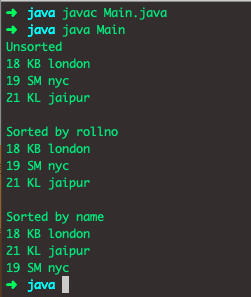# Java Comparator Interface: The Complete Guide

Java Comparator interface is used to sort an array or list of objects based on a custom order. Custom ordering of elements is imposed by implementing Comparator.compare() method in the objects.

## Java Comparator Interface

The comparator interface in Java is used to order the objects of user-defined classes. A comparator object is capable of comparing two objects of two different classes.

Java Comparator interface does the total ordering of the objects which may not have a natural ordering. For example, the natural order may be ordered by student id for a list of student objects.

But in real-life applications, we may want to sort a student’s list by their first name, date of birth, or simply any other such criteria. In such conditions, we need to use the Comparator interface.

## Use of java comparator

Sort the array or list of objects where we can not modify the object’s source code to implement a Comparable interface. Java Comparator provides multiple sorting sequences, i.e., elements can be sorted or classified based on any number, which are roll number, age, id number, etc.

## Methods of Java Comparator Interface

Method Description
public int compare(Object obj1, Object obj2) It compares the first object with the second object.
public boolean equals(Object obj) It is used to compare the current object with the specified object.
public boolean equals(Object obj) It is used to compare the current object with the specified object.

## Comparator.compare()

To empower all out requesting articles, we have to make a class that actualizes the Comparator interface. At that point, we have to supersede its compare(T o1, T o2) strategy.

It looks at its two contentions for request. It restores a negative number, zero, or a positive whole number as the principal contention is not precisely equivalent to or more noteworthy than the second.

The practitioner should likewise guarantee that the connection is transitive: ((compare(x, y)>0) &&
(compare(y, z) > 0)) suggests compare(x, z) > 0.

See the following code example.

```// Student.java

import java.util.*;

class Student {

int rollno;

public Student(int rollno, String name, String address) {
this.rollno = rollno;
this.name = name;
}

// Getters and Setters
@Override
public String toString() {
return this.rollno + " " + this.name + " " + this.address;
}
}

class Sortbyroll implements Comparator<Student> {
// Used for sorting in ascending order of
// roll number
public int compare(Student a, Student b) {
return a.rollno - b.rollno;
}
}

class Sortbyname implements Comparator<Student> {
// Used for sorting in ascending order of
// roll name
public int compare(Student a, Student b) {
return a.name.compareTo(b.name);
}
}

class Main {
public static void main(String[] args) {
ArrayList<Student> al = new ArrayList<Student>();

System.out.println("Unsorted");
for (int i = 0; i < al.size(); i++)
System.out.println(al.get(i));

Collections.sort(al, new Sortbyroll());

System.out.println("\nSorted by rollno");
for (int i = 0; i < al.size(); i++)
System.out.println(al.get(i));

Collections.sort(al, new Sortbyname());

System.out.println("\nSorted by name");
for (int i = 0; i < al.size(); i++)
System.out.println(al.get(i));
}
}```

See the output.## Collections.sort() and Arrays.sort()

1. Use Collections.sort(list, Comparator) method sort a list of objects in order imposed by provided comparator instance.
2. Use Arrays.sort(array, Comparator) method sort an array of objects in the order imposed by provided comparator instance.

That’s it for this tutorial.

This site uses Akismet to reduce spam. Learn how your comment data is processed.